astype_astype函数 - CSDN

• 关于numpy的astype（bool）和astype（int）等等 import numpy as np a=[[1,2,1],[2,3,5]] b=[[0,0,0],[2,3,5]] c=np.array(a) d=np.array(b) print(c) print(d) 就是简单的把list列表转化为数组 然后看看加了.as...
关于numpy的astype（bool）和astype（int）等等
import numpy as np
a=[[1,2,1],[2,3,5]]
b=[[0,0,0],[2,3,5]]
c=np.array(a)
d=np.array(b)
print(c)
print(d)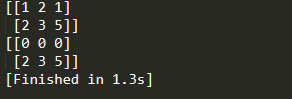就是简单的把list列表转化为数组
然后看看加了.astype(bool)是什么意思？
正如astype的中文意思，作为布尔类型，也就是true or false
代码如下
import numpy as np
a=[[1,2,1],[2,3,5]]
b=[[0,0,0],[2,3,5]]
c=np.array(a).astype(bool)
d=np.array(b).astype(bool)
print(c)
print(d)

再看看结果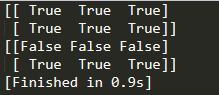这下明白了吗？0代表False   非0代表True！
那么.astype(int)是啥意思呢？
那不就是转化为整型数据吗！True和False转化为整型数据是什么样子呢？
不就是0和1嘛！
代码如下：
import numpy as np
a=[[1,2,1],[2,3,5]]
b=[[0,0,0],[2,3,5]]
c=np.array(a).astype(bool).astype(int)
d=np.array(b).astype(bool).astype(int)
print(c)
print(d)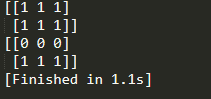看一下，是这样的吧！
那么astype(float)呢？是什么意思？不用我说你也知道了吧，那不明白看下面代码！
import numpy as np
a=[[1,2,1],[2,3,5]]
b=[[0,0,0],[2,3,5]]
c=np.array(a).astype(bool).astype(int).astype(float)
d=np.array(b).astype(bool).astype(int).astype(float)
print(c)
print(d)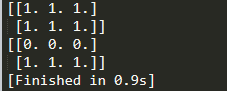希望可以帮助你


展开全文python numpy
• import numpy as np print ('生成数组时指定数据类型') arr = np.array([1, 2, 3], dtype = np.float64) print (arr.dtype) arr = np.array([1, 2, 3], dtype = np.int32) ...print ('使用astype复制数组并...
import numpy as np

print ('生成数组时指定数据类型')
arr = np.array([1, 2, 3], dtype = np.float64)
print (arr.dtype)
arr = np.array([1, 2, 3], dtype = np.int32)
print (arr.dtype)
print ( )

print ('使用astype复制数组并转换数据类型')
int_arr = np.array([1, 2, 3, 4, 5])
float_arr = int_arr.astype(np.float)
print (int_arr.dtype)
print (float_arr.dtype)
print( )

print ('使用astype将float转换为int时小数部分被舍弃')
float_arr = np.array([3.7, -1.2, -2.6, 0.5, 12.9, 10.1])
int_arr = float_arr.astype(dtype = np.int)
print (int_arr)
print( )

print ('使用astype把字符串转换为数组，如果失败抛出异常。')
str_arr = np.array(['1.25', '-9.6', '42'], dtype = np.string_)
float_arr = str_arr.astype(dtype = np.float)
print (float_arr)
print()

print ('astype使用其它数组的数据类型作为参数')
int_arr = np.arange(10)
float_arr = np.array([.23, 0.270, .357, 0.44, 0.5], dtype = np.float64)
print (int_arr.astype(float_arr.dtype))
print (int_arr, int_arr)  # astype做了复制，数组本身不变。
展开全文• astype()函数可用于转化dateframe某一列的数据类型 如下将dateframe某列的str类型转为int，注意astype()没有replace=True的用法，想要在原数据上修改，要写成如下形式。 注意只有当该列的字符串全是由纯数字构成时...
astype()函数可用于转化dateframe某一列的数据类型

如下将dateframe某列的str类型转为int，注意astype()没有replace=True的用法，想要在原数据上修改，要写成如下形式。

注意只有当该列的字符串全是由纯数字构成时才可以这样写，如果混有字母，会报错：ValueError: invalid literal for int() with base 10:

利用int()函数转字符串也类似

app_train[['uid','index']] = app_train[['uid','index']].astype('int')

isdigit()用于判断一个字符串是否由纯数字构成，如果是返回True,否则False。

承接Matlab、Python和C++的编程，机器学习、计算机视觉的理论实现及辅导，本科和硕士的均可，咸鱼交易，专业回答请走知乎，详谈请联系QQ号757160542，非诚勿扰。


展开全文1024程序员节
• ## python强制类型转换astype

万次阅读 多人点赞 2019-01-08 11:58:47
在进行将多个表的数据合并到一个表后，发现输出到...找了一些解决方法，发现用.astype('数据类型')还是挺方便的。我在输出时，将数值型的数据（int)转化成了字符串(str)。 使用方法： df.astype('数据类型') ...
在进行将多个表的数据合并到一个表后，发现输出到EXCEL表的数据发生错误，数值型数据末尾都变成了0。这是因为excel数据超过11位,自动以科学计数法显示,其最大处理精度为15位,超过15位,以后数字自动变0。找了一些解决方法，发现用.astype('数据类型')还是挺方便的。我在输出时，将数值型的数据（int)转化成了字符串(str)。

使用方法：

df.astype('数据类型')                        #改变整个df的数据类型
df['列名'].astype('数据类型')              #仅改变某一列的数据类型
先来个没有使用astype转换的输出结果：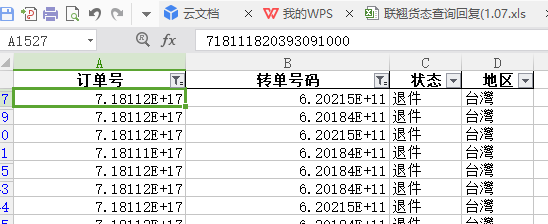可以看到 订单号 和 转单号码 都以科学计数法显示。其中订单号为18位，超过15位的显示为0。转单号码为12位，数值正确。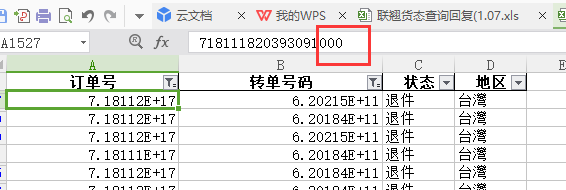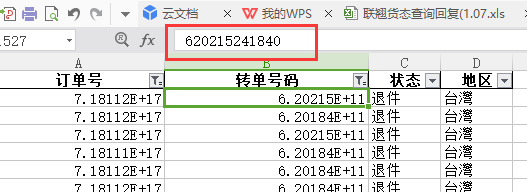插入 num=num.astype('str')这行代码，将数据转为str，输出如下：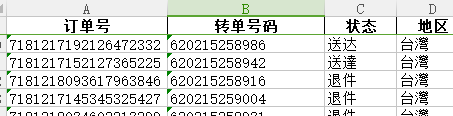全部代码如下：

#清洗台运货态表
import pandas as pd
import os
sheets=['香港','泰国','台灣']
D=[]
E=[]
for x in sheets:
data.rename(columns={'訂單號':'订单号','轉單號碼':'转单号码','狀態':'状态'},inplace=True)
d=data[['订单号','转单号码','状态']]
#len(d)输出d的行数
#d.shape#求行数
#d.shape#求列数
d['地区']=x
d.astype('object')
D.append(d)
num=pd.concat(D,axis=0)#合并list表D中的元素
num=num.astype('str')#将整个dataframe都转换为str类型
# num['订单号']=num['订单号'].astype('str')
# num['转单号码']=num['转单号码'].astype('str')
print(num.info())


除了用astype进行类型转换，也可以自定义函数来批量修改数据类型（通过int（），str()等直接转换)。还可以用Pandas的一些辅助函数(如to_numeric()、to_datetime())。知乎上也有人做了详细的描述，感谢作者。链接如下：

https://zhuanlan.zhihu.com/p/35287822
展开全文pandas 数据清洗 数据处理
• 本文介绍numpy数组中这四个方法的区别ndim、shape、dtype、astype。1.ndimndim返回的是数组的维度，返回的只有一个数，该数即表示数组的维度。2.shapeshape：表示各位维度大小的元组。返回的是一个元组。对于一维...Python Numpy shape dtype
• ## np.astype()

万次阅读 2019-07-01 20:09:42
1.作用：就是转换numpy数组的数据类型 举个例子 arr = np.arange((10)) print(arr, arr.dtype, sep="\n") =================================== [0 1 2 3 4 5 6 7 8 9] int32 #可以看到，他的...arr = arr.astype("f...
• Python中与数据类型相关函数及属性有如下三个：type/dtype/astype。 名称 描述 type() 返回参数的数据类型 dtype 返回数组中元素的数据类型 astype() 对数据类型进行转换 1.type()用于获取数据...
• 方法一ndarray.astype(np.float32)方法二float_array = np.array([1.23,3.14,5.12,3.23],dtype=float)
• python-Numpy学习之(一)ndim、shape、dtype、astype的用法 参考网址：https://blog.csdn.net/Da_wan/article/details/80518725 本文介绍numpy数组中这四个方法的区别ndim、shape、dtype、astype。 1.ndim ndim...
• ## 关于astype的坑

千次阅读 2019-03-30 21:11:40
astype并不能in place地改变一个ndarray。 例如 IN: arr = np.array([3.7,-1.2,-2.6,0.5,12.9,10.1]) OUT: array([ 3.7, -1.2, -2.6, 0.5, 12.9, 10.1]) 如果是直接输入： arr.astype(int32) 然后检查arr.dtype...numpy
• ## Numpy数据类型转换astype，dtype

万次阅读 多人点赞 2017-09-15 10:58:33
ndarray 数据类型 astype：1、查看数据类型In : arr = np.array([1,2,3,4,5])In : arr Out: array([1, 2, 3, 4, 5])// 该命令查看数据类型 In : arr.dtype Out: dtype('int64')In : float_...numpy dtype
• 标签（空格分隔）： python uint8在此输入正文8位的无符号整形数据取值范围从0到255 一singed与unsigned的区别 二float改变类型 643264 to 32 shape翻倍 改变类型 321632 to 16 shape翻倍 改变类型 32float32 to ...python
• Python中与数据类型相关函数及属性有如下三个：type/dtype/astype。 名称 描述 type() 返回参数的数据类型 dtype 返回数组中元素的数据类型 astype() 对数据类型进行转换 type()用于获取数据类型 #...Python 数据类型 type dtype
• 使用astype实现dataframe字段类型转换# -*- coding: UTF-8 -*- import pandas as pd df = pd.DataFrame([{'col1':'a', 'col2':'1'}, {'col1':'b', 'col2':'2'}])print df.dtypesdf['col2'] = df['col2'].astype('int...python dataframe
• 在有些情况下，我们需要在DataFrame类型的...Dataframe数据类型的转换需要用到astype函数。 # encoding: utf-8 import pandas as pd df = pd.DataFrame(pd.read_csv('film_log3.csv', sep=';',encoding='utf-8',
• type() 返回数据结构类型（list、dict、numpy.ndarray 等） dtype() 返回数据元素的数据类型（int、float等） 备注：1）由于 list、dict 等可以包含不同的数据类型，因此不可调用dtype()函数 ...
• NumPy最重要的一个特点就是其N维数组对象（即ndarray），该对象是一个快速而灵活的大数据集容器。你可以利用这种数组对整块数据执行一些数学运算，ndarray是一个通用的同构数据多维容器，也就是说，其中的所有元素...
• 问题出现的背景： 今天对接别人api的时候，对方引用了kubernetes下的client-go，具体信息如下： { "ImportPath": "k8s.io/client-go/pkg/api/v1", "Comment": "v4.0.0-beta.0", "Rev": "df46f7f13b3da19b90b8bgolang map index invalid
• ## numpy的astype函数

万次阅读 2017-08-22 23:27:19numpy
• import pandas as pd from matplotlib import pyplot as plt from datetime import datetime filename='sitka_weather_2014.csv' df=pd.read_csv(filename) print(df.dtypes) df[' Min Humidity']=df[' Min ....
...